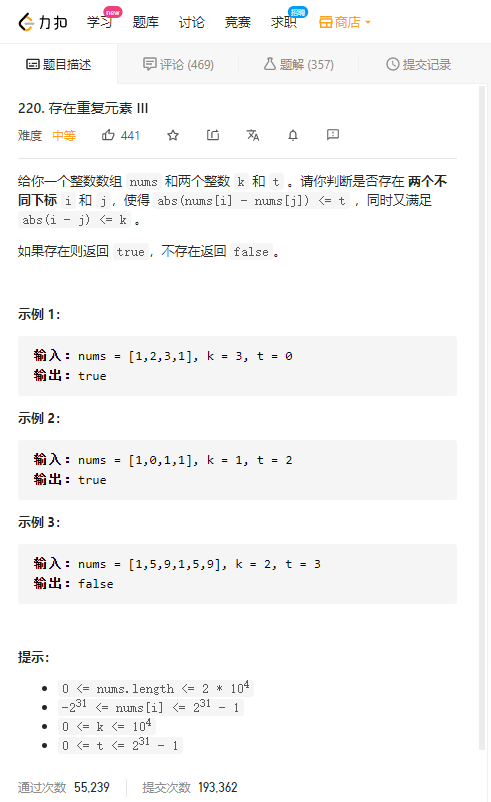# 题目描述## 方法1 滑动窗口+二分

• abs(i-j)<=k提示我们维护一个大小为k的窗口，在这个窗口内寻找满足abs(nums[i]-nums[j])<=ti,j

• 查询：能够在「有序集合」中应用「二分查找」，快速找到「小于等于 uu 的最大值」和「大于等于 u 的最小值」（即「有序集合」中的最接近 u 的数(使得abs(nums[i]-nums[j])尽可能地小 只要满足≤t存在 就返回true）。
• 插入/删除：在往「有序集合」添加或删除元素时，能够在低于线性的复杂度内完成（维持有序特性）。
class Solution {
public boolean containsNearbyAlmostDuplicate(int[] nums, int k, int t) {
int n = nums.length;
// 默认构造方法，可以传Comparator作为参数自定义排序
TreeSet<Long> ts = new TreeSet<>();
for (int i = 0; i < n; i++) {
Long u = nums[i] * 1L;
// 从 ts 中找到小于等于 u 的最大值（小于等于 u 的最接近 u 的数）
Long l = ts.floor(u);
// 从 ts 中找到大于等于 u 的最小值（大于等于 u 的最接近 u 的数）
Long r = ts.ceiling(u);
if(l != null && u - l <= t) return true;
if(r != null && r - u <= t) return true;
// 将当前数加到 ts 中，并移除下标范围不在 [max(0, i - k), i) 的数（维持滑动窗口大小为 k）
if (i >= k) ts.remove(nums[i - k] * 1L);
}
return false;
}
}


## 自定义排序用法

### 匿名函数

TreeSet<String> ts = new TreeSet<>((p,q)->(p.length()-q.length()));


### 重写Comparator的compare方法

TreeSet<String> ts = new TreeSet<>(new Comparator<String>(){
@Override
public int compare(String a,String b){
return a.length() - b.length();
}
});


## 例子：给二维数组按某列排序

	int[][] twoDims = new int[][];
Arrays.sort(twoDims,new Comparator<int[]>(){
@Override
public int compare(int[] o1, int[] o2) {
if(o1==o2){
return o1 - o2;
}
return o1 - o2;
}
});